## 机器学习：线性回归实现与应用

5870 字 · 743 阅读 · 2023 年 05 月 02 日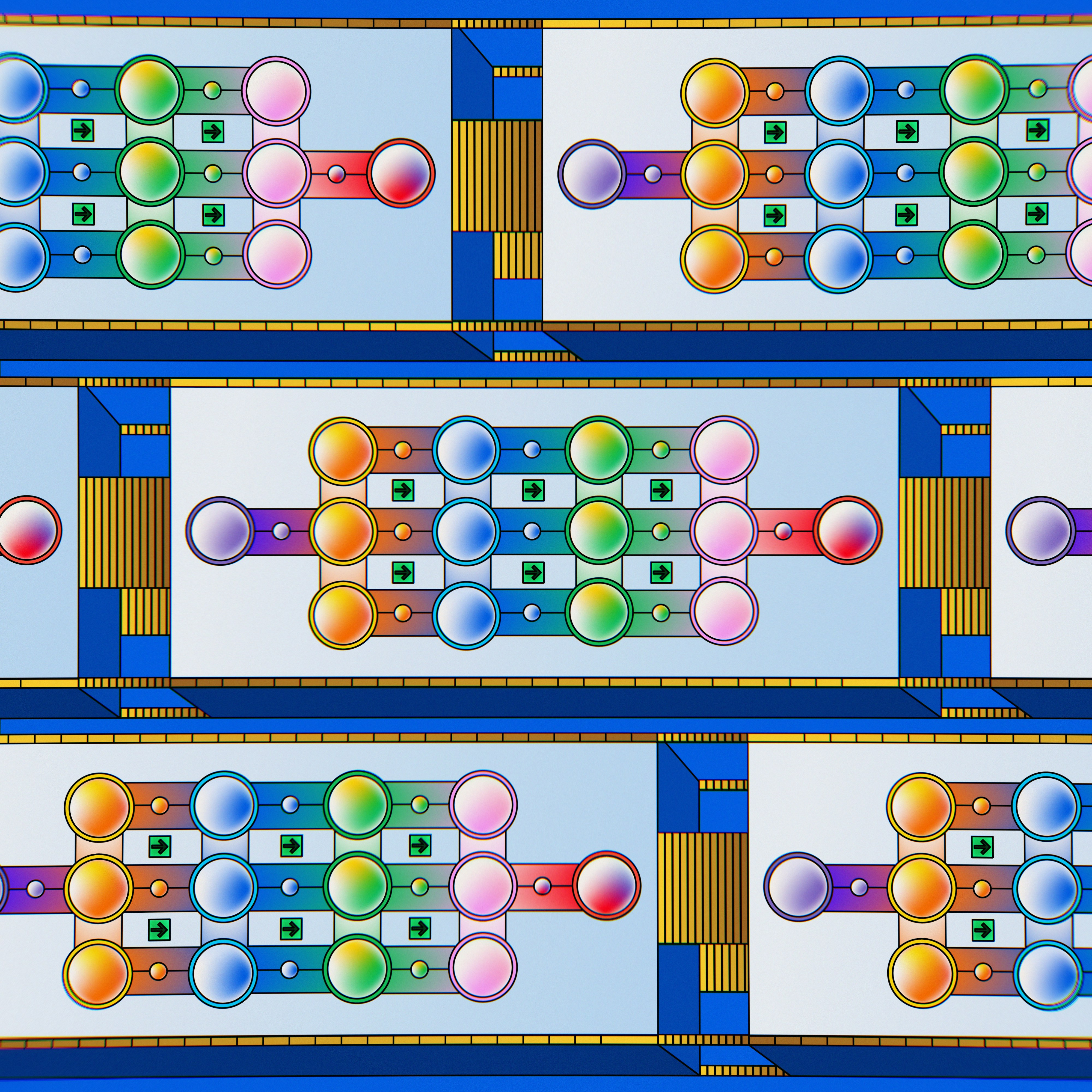• 一元线性回归
• 平方损失函数
• 最小二乘法及代数求解
• 线性回归实现
• 最小二乘法的矩阵推导

### 线性回归介绍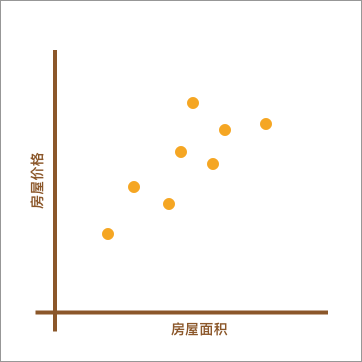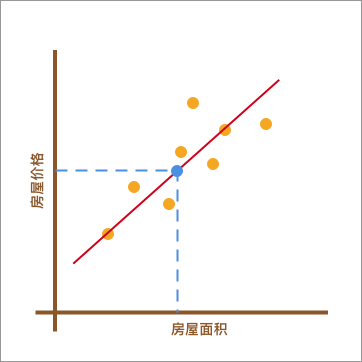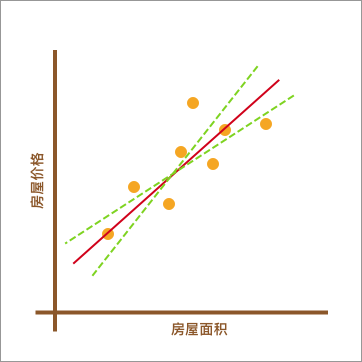### 一元线性回归

import numpy as np

x = np.array([56, 72, 69, 88, 102, 86, 76, 79, 94, 74])
y = np.array([92, 102, 86, 110, 130, 99, 96, 102, 105, 92])


# 推荐运行 Jupyter Notebook 练习代码


from matplotlib import pyplot as plt
%matplotlib inline

plt.scatter(x, y)
plt.xlabel("Area")
plt.ylabel("Price")


$$y(x, w) = w_0 + w_1x \tag{1}$$

def f(x, w0, w1):
y = w0 + w1 * x
return y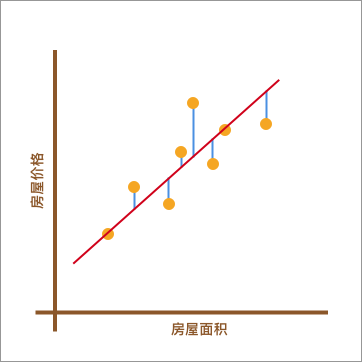### 平方损失函数

$$y_{i}-(w_0 + w_1x_{i}) \tag{2}$$

$$\sum\limits_{i = 1}^n {{{(y_{i}-(w_0 + w_1x_{i}))}}} \tag{3}$$

$$\sum\limits_{i = 1}^n {{{(y_{i}-(w_0 + w_1x_{i}))}}^2} \tag{4}$$

def square_loss(x, y, w0, w1):
loss = sum(np.square(y - (w0 + w1*x)))
return loss


### 最小二乘法及代数求解

$$f = \sum\limits_{i = 1}^n {{{(y_{i}-(w_0 + w_1x_{i}))}}^2} \tag{5}$$

$$\frac{\partial f}{\partial w_{0}}=-2(\sum_{i=1}^{n}{y_i}-nw_{0}-w_{1}\sum_{i=1}^{n}{x_i}) \tag{6a}$$
$$\frac{\partial f}{\partial w_{1}}=-2(\sum_{i=1}^{n}{x_iy_i}-w_{0}\sum_{i=1}^{n}{x_i}-w_{1}\sum_{i=1}^{n}{x_i}^2) \tag{6b}$$

$$w_{1}=\frac {n\sum_{}^{}{x_iy_i}-\sum_{}^{}{x_i}\sum_{}^{}{y_i}} {n\sum_{}^{}{x_i}^2-(\sum_{}^{}{x_i})^2} \tag{7b}$$
$$w_{0}=\frac {\sum_{}^{}{x_i}^2\sum_{}^{}{y_i}-\sum_{}^{}{x_i}\sum_{}^{}{x_iy_i}} {n\sum_{}^{}{x_i}^2-(\sum_{}^{}{x_i})^2} \tag{7b}$$

### 线性回归 Python 实现

def w_calculator(x, y):
n = len(x)
w1 = (n*sum(x*y) - sum(x)*sum(y))/(n*sum(x*x) - sum(x)*sum(x))
w0 = (sum(x*x)*sum(y) - sum(x)*sum(x*y))/(n*sum(x*x)-sum(x)*sum(x))
return w0, w1


w_calculator(x, y)


w0 = w_calculator(x, y)
w1 = w_calculator(x, y)

square_loss(x, y, w0, w1)


x_temp = np.linspace(50, 120, 100)  # 绘制直线生成的临时点

plt.scatter(x, y)
plt.plot(x_temp, x_temp*w1 + w0, 'r')


f(150, w0, w1)


### 线性回归 scikit-learn 实现

sklearn.linear_model.LinearRegression(fit_intercept=True, normalize=False, copy_X=True, n_jobs=1)



- fit_intercept: 默认为 True，计算截距项。

- normalize: 默认为 False，不针对数据进行标准化处理。

- copy_X: 默认为 True，即使用数据的副本进行操作，防止影响原数据。

- n_jobs: 计算时的作业数量。默认为 1，若为 -1 则使用全部 CPU 参与运算。


from sklearn.linear_model import LinearRegression

# 定义线性回归模型
model = LinearRegression()
model.fit(x.reshape(len(x), 1), y)  # 训练, reshape 操作把数据处理成 fit 能接受的形状

# 得到模型拟合参数
model.intercept_, model.coef_


model.predict([])


### 最小二乘法的矩阵推导及实现

$$\left[ \begin{array}{c}{1, x_{1}} \\ {1, x_{2}} \\ {\cdots} \\ {1, x_{9}} \\ {1, x_{10}}\end{array}\right] \left[ \begin{array}{c}{w_{0}} \\ {w_{1}}\end{array}\right] = \left[ \begin{array}{c}{y_{1}} \\ {y_{2}} \\ {\cdots} \\ {y_{9}} \\ {y_{10}}\end{array}\right] \Rightarrow \left[ \begin{array}{c}{1,56} \\ {1,72} \\ {\cdots} \\ {1,94} \\ {1,74}\end{array}\right] \left[ \begin{array}{c}{w_{0}} \\ {w_{1}}\end{array}\right]=\left[ \begin{array}{c}{92} \\ {102} \\ {\cdots} \\ {105} \\ {92}\end{array}\right] \tag{8a}$$

$$y(x, w) = XW \tag{8b}$$

$(8)$ 式中，$W$ 为：

$$\begin{bmatrix}w_{0}\\ w_{1}\end{bmatrix}$$

$$\begin{bmatrix}1, x_{1}\\ 1, x_{2}\\ \cdots\\ 1, x_{9}\\ 1, x_{10}\end{bmatrix}$$

$$f = \sum\limits_{i = 1}^n {{{(y_{i}-(w_0 + w_1x_{i}))}}}^2 =(y-XW)^T(y-XW)\tag{9}$$

$$f = y^{T}y - y^{T}(XW) - (XW)^{T}y + (XW)^{T}(XW) \tag{10}$$

$$f = y^{T}y - (XW)^{T}y - (XW)^{T}y + (XW)^{T}(XW)\\ = y^{T}y - 2 (XW)^{T}y + (XW)^{T}(XW) \tag{11}$$

$$\frac{\partial f}{\partial W}=2X^TXW-2X^Ty=0 \tag{12}$$

$$W=(X^TX)^{-1}X^Ty \tag{13}$$

def w_matrix(x, y):
w = (x.T * x).I * x.T * y
return w


x = np.matrix([[1, 56], [1, 72], [1, 69], [1, 88], [1, 102],
[1, 86], [1, 76], [1, 79], [1, 94], [1, 74]])
y = np.matrix([92, 102, 86, 110, 130, 99, 96, 102, 105, 92])

w_matrix(x, y.reshape(10, 1))


### 数据集介绍及划分

import pandas as pd

"https://labfile.oss.aliyuncs.com/courses/1081/course-5-boston.csv")


df.head()


• CRIM: 城镇犯罪率。
• ZN: 占地面积超过 2.5 万平方英尺的住宅用地比例。
• INDUS: 城镇非零售业务地区的比例。
• CHAS: 查尔斯河是否经过 (=1 经过，=0 不经过)。
• NOX: 一氧化氮浓度（每 1000 万份）。
• RM: 住宅平均房间数。
• AGE: 所有者年龄。
• DIS: 与就业中心的距离。
• RAD: 公路可达性指数。
• TAX: 物业税率。
• PTRATIO: 城镇师生比例。
• BLACK: 城镇的黑人指数。
• LSTAT: 人口中地位较低人群的百分数。
• MEDV: 城镇住房价格中位数。

features = df[['crim', 'rm', 'lstat']]
features.describe()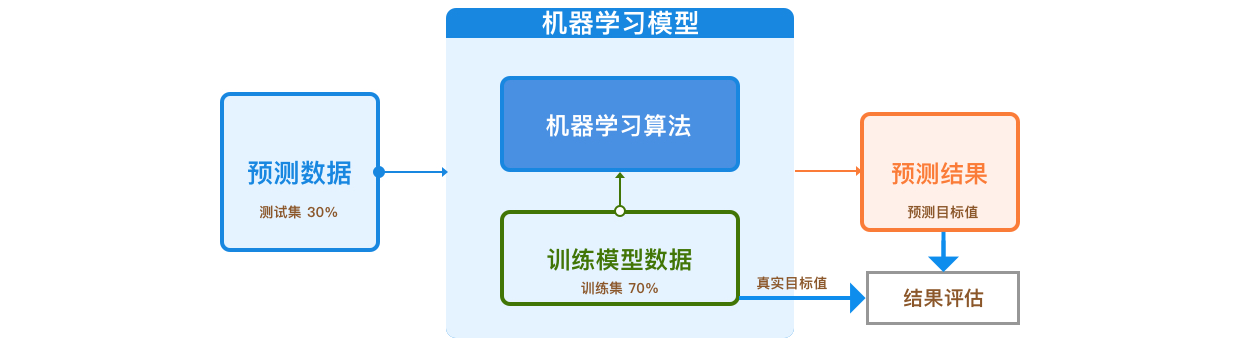target = df['medv']  # 目标值数据

split_num = int(len(features)*0.7)  # 得到 70% 位置

X_train = features[:split_num]  # 训练集特征
y_train = target[:split_num]  # 训练集目标

X_test = features[split_num:]  # 测试集特征
y_test = target[split_num:]  # 测试集目标


### 构建和训练模型

model = LinearRegression()  # 建立模型
model.fit(X_train, y_train)  # 训练模型
model.coef_, model.intercept_  # 输出训练后的模型参数和截距项


$$f = 0.6997 * x_{1} + 10.1356 * x_{2} - 0.2053 * x_{3} - 38 \tag{14}$$

preds = model.predict(X_test)  # 输入测试集特征进行预测
preds  # 预测结果


$$\textrm{MAE}(y, \hat{y} ) = \frac{1}{n}\sum_{i=1}^{n}{|y_{i}-\hat y_{i}|}\tag{15}$$

def mae_value(y_true, y_pred):
n = len(y_true)
mae = sum(np.abs(y_true - y_pred))/n
return mae


$$\textrm{MSE}(y, \hat{y} ) = \frac{1}{n} \sum_{i=1}^{n} (y_i - \hat{y_i})^{2}\tag{16}$$

def mse_value(y_true, y_pred):
n = len(y_true)
mse = sum(np.square(y_true - y_pred))/n
return mse


mae = mae_value(y_test.values, preds)
mse = mse_value(y_test.values, preds)

print("MAE: ", mae)
print("MSE: ", mse)


### 系列文章•